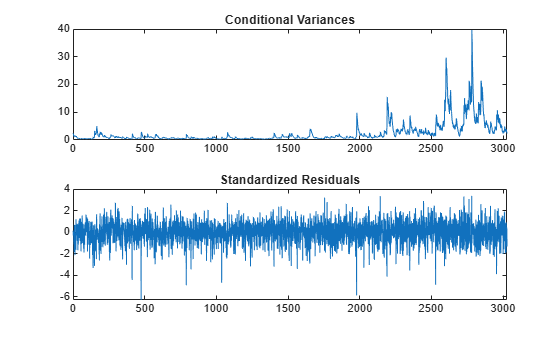# Estimate Conditional Mean and Variance Model

This example shows how to estimate a composite conditional mean and variance model using `estimate`.

Load the NASDAQ data included with Econometrics Toolbox™. Convert the daily close composite index series to a return series. For numerical stability, convert the returns to percentage returns.

```load Data_EquityIdx nasdaq = DataTable.NASDAQ; r = 100*price2ret(nasdaq); T = length(r);```

### Create Model Template

Create an AR(1) and GARCH(1,1) composite model, which has the form

`${r}_{t}=c+{\varphi }_{1}{r}_{t-1}+{\epsilon }_{t},$`

`${\sigma }_{t}^{2}=\kappa +{\gamma }_{1}{\sigma }_{t-1}^{2}+{\alpha }_{1}{\epsilon }_{t-1}^{2},$`

where ${\epsilon }_{t}={\sigma }_{t}{z}_{t}$ and ${z}_{t}$ is an iid standardized Gaussian process.

`VarMdl = garch(1,1)`
```VarMdl = garch with properties: Description: "GARCH(1,1) Conditional Variance Model (Gaussian Distribution)" SeriesName: "Y" Distribution: Name = "Gaussian" P: 1 Q: 1 Constant: NaN GARCH: {NaN} at lag  ARCH: {NaN} at lag  Offset: 0 ```
`Mdl = arima('ARLags',1,'Variance',VarMdl)`
```Mdl = arima with properties: Description: "ARIMA(1,0,0) Model (Gaussian Distribution)" Distribution: Name = "Gaussian" P: 1 D: 0 Q: 0 Constant: NaN AR: {NaN} at lag  SAR: {} MA: {} SMA: {} Seasonality: 0 Beta: [1×0] Variance: [GARCH(1,1) Model] ```

`Mdl` is an `arima` model template for estimation. `NaN`-valued properties of `Mdl` and `VarMdl` correspond to unknown, estimable coefficients and variance parameters of the composite model.

### Estimate Model Parameters

Fit the model to the return series `r` by using `estimate`.

`EstMdl = estimate(Mdl,r);`
``` ARIMA(1,0,0) Model (Gaussian Distribution): Value StandardError TStatistic PValue ________ _____________ __________ __________ Constant 0.072632 0.018047 4.0245 5.7087e-05 AR{1} 0.13816 0.019893 6.945 3.7848e-12 GARCH(1,1) Conditional Variance Model (Gaussian Distribution): Value StandardError TStatistic PValue ________ _____________ __________ __________ Constant 0.022377 0.0033201 6.7399 1.5851e-11 GARCH{1} 0.87312 0.0091019 95.927 0 ARCH{1} 0.11865 0.008717 13.611 3.434e-42 ```

`EstMdl` is a fully specified `arima` model.

The estimation display shows the five estimated parameters and their corresponding standard errors (the AR(1) conditional mean model has two parameters, and the GARCH(1,1) conditional variance model has three parameters).

The fitted model (`EstMdl`) is

`${r}_{t}=0.073+0.138{r}_{t-1}+{\epsilon }_{t},$`

`${\sigma }_{t}^{2}=0.022+0.873{\sigma }_{t-1}^{2}+0.119{\epsilon }_{t-1}^{2}.$`

All $\mathit{t}$ statistics are greater than 2, which suggests that all parameters are statistically significant.

Dynamic models require presample observations, with which to initialize the model. If you do not specify presample observations, `estimate` generates them by default.

### Infer Conditional Variances and Residuals

Infer and plot the conditional variances and standardized residuals. Output the loglikelihood objective function value.

```[res,v,logL] = infer(EstMdl,r); figure subplot(2,1,1) plot(v) xlim([0,T]) title('Conditional Variances') subplot(2,1,2) plot(res./sqrt(v)) xlim([0,T]) title('Standardized Residuals')```The conditional variances increase after observation 2000. This result corresponds to the increased volatility seen in the original return series.

The standardized residuals have more large values (larger than 2 or 3 in absolute value) than expected under a standard normal distribution. This result suggests a Student's $\mathit{t}$ distribution can be more appropriate for the innovation distribution.

### Fit Model With t Innovation Distribution

Create a model template from `Mdl`, and specify that its innovations have a Student's $\mathit{t}$ distribution.

```MdlT = Mdl; MdlT.Distribution = 't';```

`MdlT` has one additional parameter estimate: the $\mathit{t}$ distribution degrees of freedom.

Fit the new model to the NASDAQ return series. Specify an initial value for the variance model constant term.

```Variance0 = {'Constant0',0.001}; EstMdlT = estimate(MdlT,r,'Variance0',Variance0);```
``` ARIMA(1,0,0) Model (t Distribution): Value StandardError TStatistic PValue ________ _____________ __________ __________ Constant 0.093488 0.016694 5.6002 2.1414e-08 AR{1} 0.13911 0.018857 7.3771 1.6175e-13 DoF 7.4775 0.88261 8.472 2.4126e-17 GARCH(1,1) Conditional Variance Model (t Distribution): Value StandardError TStatistic PValue ________ _____________ __________ __________ Constant 0.011246 0.0036305 3.0976 0.0019511 GARCH{1} 0.90766 0.010516 86.315 0 ARCH{1} 0.089897 0.010835 8.2966 1.0712e-16 DoF 7.4775 0.88261 8.472 2.4126e-17 ```

The coefficient estimates between `EstMdl` and `EstMdlT` are slightly different. The degrees of freedom estimate is relatively small (about 8), which indicates a significant departure from normality.

### Compare Model Fits

Summarize the estimated models. From the summaries, obtain the number of estimated parameters and the loglikelihood objective function value from the second fit.

```Summary = summarize(EstMdl); SummaryT = summarize(EstMdlT); numparams = Summary.NumEstimatedParameters; numparamsT = SummaryT.NumEstimatedParameters; logLT = SummaryT.LogLikelihood;```

Compare the two model fits (Gaussian and $\mathit{t}$ innovation distribution) using the Akaike information criterion (AIC) and Bayesian information criterion (BIC).

`[numparams numparamsT]`
```ans = 1×2 5 6 ```
`[aic,bic] = aicbic([logL logLT],[numparams numparamsT],T)`
```aic = 1×2 103 × 9.4929 9.3807 ```
```bic = 1×2 103 × 9.5230 9.4168 ```

The first model has six fitted parameters, whereas the second model has six (because it contains the $\mathit{t}$ distribution degrees of freedom). Despite this difference, both information criteria favor the model with the Student's $\mathit{t}$ innovation distribution because it yields smaller AIC and BIC values than the model with Gaussian innovations.